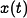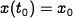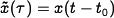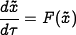# Difference between revisions of "Errata: In Exercise 4.1, x 0 should not be subtracted from time-shifted solution"

Show that if we have a solution of the differential equation (4.x) given bywith initial condition, thenis a solution of the differential equation